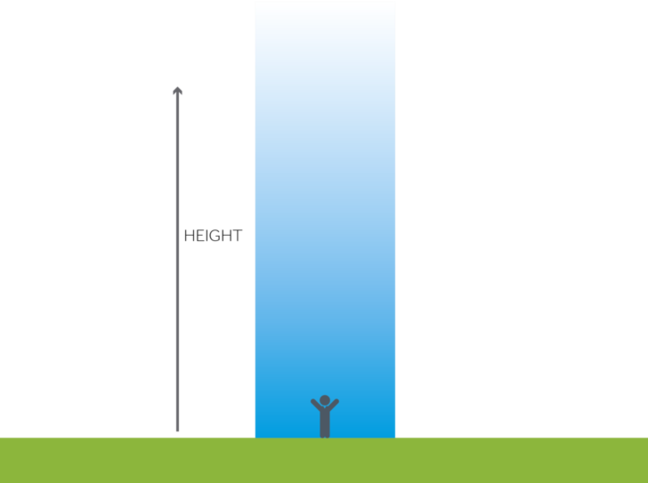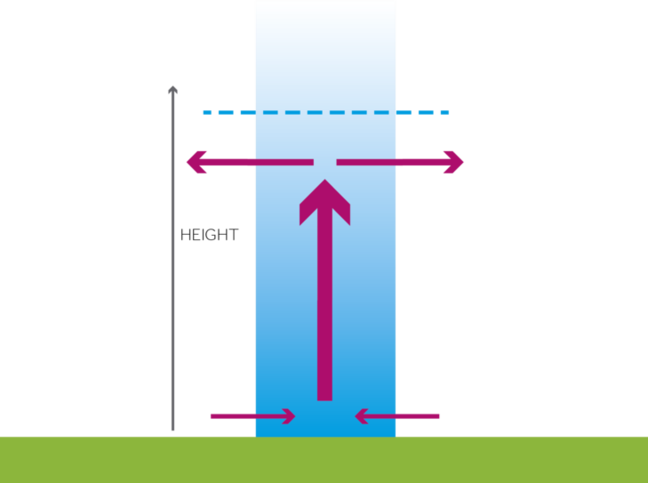## Want to keep learning?

This content is taken from the University of Reading & The Royal Meteorological Society 's online course, Come Rain or Shine: Understanding the Weather. Join the course to learn more.
1.12

# Pressure and wind

In Step 1.10 you explored why cloud and rain are associated with a depression. But why are they low pressure systems, and how do we get wind?

First of all let’s consider atmospheric pressure. This is a measure of how much air is in the column above your head (see Figure 1) and is typically around 1000hPa (or millibars), equivalent to about 10m of water.##### Figure 1: Air pressure is a measure of how much air is above your head © Dr Peter Inness

Near the centre of a depression, air is rising.

The tropopause, the boundary between the troposphere (the bottom 10km or so of the atmosphere) and the stratosphere above, acts as a lid to vertical motion, so the air spreads out horizontally at this level (you’ll learn more about lapse rates and stability in Week 2). Near the surface, air converges into the column to replace the air being lost through rising motion. If more air diverges than converges, we end up with less air in the column and the air pressure falls (Figure 2).##### Figure 2: Near the centre of a depression, more air is spreading out at the top than is coming in at the bottom. © Dr Peter Inness

So we end up with low pressure in our depression, and higher pressure around it.

With divergence at the top of the column and convergence at the bottom you might expect the mass of the column, and hence the pressure, to remain unchanged as one would be compensating exactly for the other. However, near the surface friction is acting on the air parcels as they move, slowing them down. This means that the convergence near the surface is always a little less than the divergence aloft and so the mass of the column decreases and the pressure falls. This will be the case until the divergence at the top of the column weakens or stops completely.

Now consider two buckets of water, one containing more water than the other. You know that, if you are able to remove the wall between the buckets, the water will flow between them until the levels are the same (Figure 3).##### Figure 3: If you have two containers, one with more water in and another with less, and you remove the barrier between them, then the water quickly flows to even out the level.

Exactly the same happens in the atmosphere; if there is more air in one place (high pressure), and less elsewhere (low pressure) then the air moves from high to low. The stronger the pressure gradient the bigger the difference between high and low pressure; or the closer the pressure contours are to each other, the faster the wind. The absolute value of pressure in the centre of the depression is less important than the difference in pressure between the centre and the edge of the system.

However, we live on a rotating, spherical planet. So we also need to take the Coriolis force, created by the rotation of the Earth, into account. Let’s take a closer look at this in the next Step.